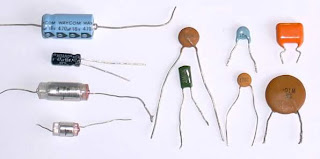## Saturday, 25 August 2012

### COLOUR CODING OF CAPACITORSIn electronic field the most important one thing is knowing componets and the values of that conponent. In this we going to familiarising with calculation of capacitance of capacitor .
In small value capacitors like mica capacitors , value of capacitors will be written on it in a coded form. The method of reading the value is explained below.

1. If the number written on the capacitor is greater than one, the value will be in pF. Otherwise , it will be in μF , For example
10 means 10pF and 0.1 means 0.1 μF .

2. If the there are three digits in the number, the third number indicates the number of zeros to be put after two digits and the value will be in pF. 104 means 10,0000pF or 0.1μF.

3. If the letter k follows the digits, the value will be in kpF. 10k means 10kpF or 0.01 μF.

4. If the letter is 'n' or 'M' the value will be that much nano farads respectivly. 47n means 47nF and 47M means 47 μF.

5. If the letter n,M or k is between two numerals, the value of the capacitor can be obtained by putting a decimal in place of the ietter and multiplying by the factor nF, μF, or kpF respectivly. 4k7 means 4.7 kpF and 2M2 means 2.2μF.

6. If the letter k or M follows the three digit number , it implies the tolerance value 10% and 20% respectivly.Colour CodingDifferent colour coding methods are used for representing capacitance value. Here im explain a simple way to find capacitance. We know the five colour band system. It is very popular. The first band gives the first significanf digit second band gives the second significant digit, third band gives the multiplier fourth gives the tolerance in percentage and fifth gives the working voltage. The values can seen in the below table. Use of this we cab calculate the capacitance of capacitor.#### 1 comment:

1.Nice Blog, Keep it up......

feel free to check my Electronics Circuits Related Blog:-
MY CIRCUITS 9